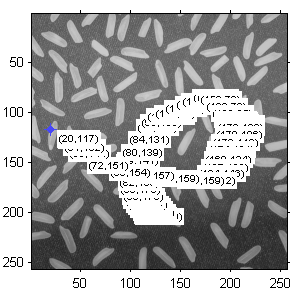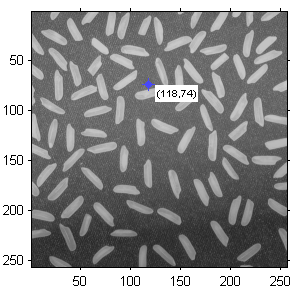# Solving a MATLAB bug by subclassing

I would like to welcome guest blogger Matthew Whitaker. Many of Matt’s CSSM submissions offer important insight of internal Matlab functionality. As shall be seen by today’s article and some future submissions, Matt has plenty to share vis-a-vis Matlab’s undocumented functionality.

In my day-to-day work I make extensive use of MATLAB’s Image Processing Toolbox (IPT). One area of the toolbox that has seen considerable change over the last few releases has been the development of a set of modular tools to aid in GUI design for image processing applications. In this article, I examine a bug in one of those tools to illustrate how we can use the power of subclassing these objects (using an undocumented property) to design a simple and effective workaround.

### The problem

The problem arose as I was refactoring some code that was written in R2006b to R2009b. The code in question uses the impoint tool on an image along with an associated text object that moves with the point to display information as it is dragged around the image. At the time of the R2006b release the impoint tool was written as an API. In R2006b the call to impoint returns a handle to an hggroup containing a structure of function handles in its application data under the tag ‘API’. This programming pattern was common before the advent of the new class syntax in MATLAB version 7.6 (R2008a).

Here is an example of how impoint would be used in R2006b:

```function impointBehavior_R2006b %IMPOINTBEHAVIOR_R2006B shows how impoint would be used in R2006b %Note: RUN UNDER R2006B (will run under R2009b but actually uses %classdef impoint so it will show the same issue)   % Display the image in a figure window figure; imshow('rice.png');   % In R2006b calling impoint returns the hggroup handle h = impoint(gca,100,200);   % In 2006b iptgetapi returns a structure of function handles api = iptgetapi(h);   % Add a new position callback to set the text string api.addNewPositionCallback(@newPos_Callback);   % Construct boundary constraint function so we can't go outside the axes fcn = makeConstrainToRectFcn('impoint',get(gca,'XLim'),get(gca,'YLim')); api.setDragConstraintFcn(fcn);   % Fire callback so we get initial text newPos_Callback(api.getPosition());   function newPos_Callback(newPos) % Display the current point position in a text label api.setString(sprintf('(%1.0f,%1.0f)',newPos(1),newPos(2))); end %newPos_Callback   end %impointBehavior_R2006b```

The code above, when run in R2006b, produces the desired behavior of displaying a text object containing the point coordinates that moves around with the point as it is dragged around the axes.

In R2009b, impoint is now a true MATLAB class using the new classdef syntax, so I wanted to update the existing code. Initially this appeared to be a straightforward translation of the code to make use of the new impoint class syntax. The first attempt to rewrite the code was:

```function impointBehavior_R2009b %IMPOINTBEHAVIOR_R2009B shows the undesirable behavior when %using the setString method in R2009b.   % Display the image in a figure window figure; imshow('rice.png'); h = impoint(gca,100,200);   % Add a new position callback to set the text string h.addNewPositionCallback(@newPos_Callback);   % Construct boundary constraint function so we can't go outside the axes fcn = makeConstrainToRectFcn('impoint',get(gca,'XLim'),get(gca,'YLim'));   % Enforce boundary constraint function h.setPositionConstraintFcn(fcn);   % Fire callback so we get initial text newPos_Callback(h.getPosition());   function newPos_Callback(newPos) % Display the current point position in a text label h.setString(sprintf('(%1.0f,%1.0f)',newPos(1),newPos(2))) end %newPos_Callback   end %impointBehavior_R2009b```

Unfortunately, when this code is run, dragging the mouse around the axes produces a trail of labels as shown below:Before - buggy behavior

Opening up impoint.m in the editor and tracing the code revealed that impoint‘s setString method creates a new text object each time it is used. I reported this to MATLAB and the bug is now documented on the MathWorks Support site (574846).

### The solution

So how do we work around this bug to get to the behavior we want? One solution would be to rewrite the offending MATLAB code but this is somewhat risky in terms of maintainability and compatibility.

A more elegant solution is to subclass the impoint class and substitute the setString behavior we want. Looking at the impoint code we find that impoint is a subclass of imroi. In the imroi property declarations we see a number of undocumented properties that are protected. We can access these properties in a subclass but not outside the class. One of these undocumented properties is h_group which is an hggroup that contains the handle graphic objects that make up the impoint on the screen. The label, when created, becomes part of this hggroup with its Tag property set to ‘label’. When performing the setString method the behavior we want to see is that if the text object exists we want to update its String property. If it does not exist we want it to perform its existing functionality:

```classdef impointtextupdate < impoint %IMPOINTTEXTUPDATE subclasses impoint to override the setString %method of impoint so that it does not create a new text object %each time it is called.   methods function obj = impointtextupdate(varargin) obj = obj@impoint(varargin{:}); end %impointtextupdate   function setString(obj,str) %override impoint setString   %check to see if there is already a text label label = findobj(obj.h_group,'Type','text','Tag','label');   if isempty(label) %do the default behavior setString@impoint(obj,str); else %update the existing tag set(label(1),'String',str); end %if end %setString   end %methods end %impointtextupdate```

Substituting calls to impoint with the new impointupdatetext subclass now produces the desired effect as shown below:After - expected behavior

### Conclusions

This case illustrates a couple of points:

• Much of the existing code in the MATLAB toolboxes is being updated to the new object oriented syntax. This presents many opportunities to easily and elegantly modify the default behavior without modifying provided toolbox code In the example above we retain all the desirable behavior of impoint while overriding the undesirable behavior.
• Many of the properties and methods in the provided toolbox objects are hidden or protected and are undocumented. It takes some simple detective work to find these out through examining the code. MATLAB is very generous in providing much of the existing code openly. Open the functions and classes you use in the editor to really find out how they work. Over the years I’ve learned and adopted a lot of useful MATLAB programming patterns by examining the code in the various toolboxes (there are a few coding horrors as well!).

I hope to explore some other under-documented features of the IPT and other toolboxes in future posts.Print

### 4 Responses to Solving a MATLAB bug by subclassing

1.Foster says:

Good post, looking forward to more IPT-related posts in the future.
I’m new to this ‘detective work’ and have a quick q.. I’m using the impoly function, and trying to make the vertices smaller. I made the lines thinner by editing the (undocumented) polygonSymbol, but I can’t find the bit to change the points…keep getting dead ends. I’ve figured it uses impoint but I’m stuck on accessing the handle objects. Any *ahem* pointers?

•Matt Whitaker says:

Hi Foster,
This is another good situation for subclassing.
Here is a pretty minimal class for setting the vertex size. Note it does not handle the situation of adding a new vertex or changing vertex color after creation. For now you can just call the setVertexSize method after doing one of those operations. That’s slightly more sophisticated and may be worth a complete blog entry.

```classdef impoly_vertex < impoly   properties (GetAccess = public, SetAccess = private) vertexCrossSize; vertexDotSize; end %properties   methods function obj = impoly_vertex(crossSize,dotSize,varargin) if nargin < 2 error('impoly_vertex:InsufficientArguments','Both the cross and dot size must be passed as constructor arguments'); end %if obj = obj@impoly(varargin{:}); obj.parseSizeInputs(crossSize,dotSize); obj.setVertexSize; end %impoly_vertex   function setVertexSize(obj) crossChildren = findobj(obj.h_group,'Type','line','Marker','+'); dotChildren = findobj(obj.h_group,'Type','line','Marker','O'); set(crossChildren,'MarkerSize',obj.vertexCrossSize); set(dotChildren,'MarkerSize',obj.vertexDotSize); end   function setCrossSize(obj,cs) obj.parseSizeInputs(cs,obj.vertexDotSize); obj.setVertexSize; end %setCrossSize   function setDotSize(obj,ds) obj.parseSizeInputs(obj.vertexCrossSize,ds); obj.setVertexSize; end %setCrossSize end   methods (Access = protected) function parseSizeInputs(obj,cs,ds) validateattributes(cs,{'numeric'},{'scalar','nonnegative','real'}); validateattributes(ds,{'numeric'},{'scalar','nonnegative','real'}); obj.vertexCrossSize = cs; obj.vertexDotSize = ds; end %parseSizeInputs end %private Methods   end %impoly_vertex```

Here we let it draw the polygon then find the vertices and change the marker size of the lines that make them up. Usage as follows:

```figure, imshow('gantrycrane.png'); %about half the regular vertex size h = impoly_vertex(4.5,2.25,gca, [188,30; 189,142; 93,141; 13,41; 14,29]); setColor(h,'yellow'); h.setVertexSize; %need to reset manually for this version addNewPositionCallback(h,@(p) title(mat2str(p,3))); fcn = makeConstrainToRectFcn('impoly',get(gca,'XLim'),... get(gca,'YLim')); setPositionConstraintFcn(h,fcn);```

A couple more points:

I really try to avoid editing any of the supplied code as it becomes a maintenance headache when you change versions.

Also a useful detective tool is the metaclass class

So mc = ?impoly gives you all sorts of useful info to help design you subclass.

Hope this Helps
Matt W

2.sumaia says:

I am trying to run an example that uses validateattributes. My matlab is an older version and doesn’t recognise this function. Is it possible to load this function to my matlab and if so can you tell me how. I also need the function list.
Many thanks

•Yair Altman says:

@sumaia – validateattributes simply ensures that the specified input variable is of the expected type. You can make these checks yourself, without really needing to use validateattributes. Or, if you are confident that the variables are of the expected type, you can skip these checks altogether.